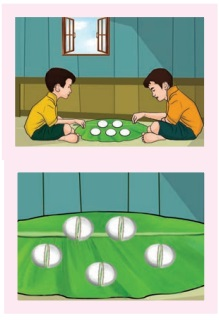Home | | Maths 6th Std | Improper and Mixed Fractions

# Improper and Mixed Fractions

Quotient + [ Remainder / Divisor ] = Quotient [ Remainder / Divisor ].

Improper and Mixed Fractions

Iniyan had 5 idlis for his breakfast. When he was about to eat, his friend Abdul came. He wanted to share it equally with his friend Abdul. Both of them have taken 2 each and 1/2 of the remaining idli.Each one has eaten 2 full idlis and 1/2 idli. This can be represented as 2 + 1/2 = 2 1/2. This representation is called a Mixed fraction. Thus, a mixed fraction is the sum of a whole number and a proper fraction. Also we can express an improper fraction as a mixed fraction by dividing the numerator by denominator to get quotient and remainder. Thus, any mixed fraction can be written as

Quotient + [ Remainder / Divisor ] = Quotient [ Remainder / Divisor ].Another way to share these idlis is as follows: Now can you see how many halves are there in 5 idlis. There are 10 halves. If we share these 1/2 idlis each time, then Iniyan  and Abdul has eaten 5 halves each. That iswhich is same as. Thus, any improper fraction can be written as mixed fraction as

Improper fraction = [ (Whole number × Denominator) + Numerator ] / DenominatorTry these

1. Complete the following table. The first one is done for you.Example 10

Convert 5 3/7 into an improper fraction.

Solution

Improper fraction = [ (Whole number ×Denominator) + Numerator ] / DenominatorThinkExample 11

Convert 17/3 into a mixed fraction.

SolutionTry these

i) Convent 3 1/3 into improper fraction

3 1/3 improper fraction 10/3

ii) Convent 45/7  into improper fraction

Mixed fraction of 45/7 = 6 3/7

Tags : Fractions | Term 3 Chapter 1 | 6th Maths , 6th Maths : Term 3 Unit 1 : Fractions
Study Material, Lecturing Notes, Assignment, Reference, Wiki description explanation, brief detail
6th Maths : Term 3 Unit 1 : Fractions : Improper and Mixed Fractions | Fractions | Term 3 Chapter 1 | 6th Maths